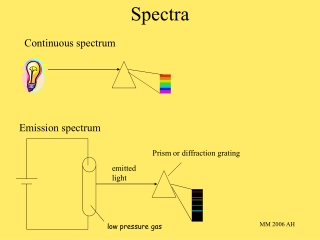DownloadDownload PresentationSpectra

# Spectra

Télécharger la présentation## Spectra

- - - - - - - - - - - - - - - - - - - - - - - - - - - E N D - - - - - - - - - - - - - - - - - - - - - - - - - - -
##### Presentation Transcript

1. Prism or diffraction grating emitted light low pressure gas Continuous spectrum Spectra Emission spectrum MM 2006 AH

2. 500 600 550 650 Emission spectrum of neon , nm MM 2006 AH

3. , nm 656 486 434 410 Emission spectrum of hydrogen The Balmer series E = Lh E = L h c l c = ln • energy emitted when excited electrons fall back to a lower energy level • frequency of line due to difference in energy between 2 electron energy levels MM 2006 AH

4. E3 E2 E3 E1 + E2 E1 Energy levels DEb = E3 - E2 = hb DEa = E3 - E1 = ha • frequency of lines in emission spectrum fixed • energy between levels fixed • energy of electrons fixed - quantised - photon emitted when electron moves to lower energy level MM 2006 AH

5. Full emission spectrum of hydrogen P7 & 8 LTS MM 2006 AH

6. , nm 121.6 102.6 97.3 91.2 convergence limit The Lyman series p9 LTS Q MM 2006 AH

7. Bohr’s theory • the hydrogen atom’s electron exists only in certain definite energy levels • the electron changes energy levels when a photon is absorbed or emitted • the energy of the photon equals the difference between the two energy levels • DE = hn. Quanta - fixed quantities of energy possessed by electrons MM 2006 AH

8. Quantum mechanics electrons - wave-particle duality 4 quantum numbers define energy of an electron electrons arranged in shells shells described by principal quantum number, n n = 1, closest to nucleus; second shell n = 2 the higher n, the higher the potential energy associated the shell and the further from the nucleus the electron likely to be found Spectra show doublets and triplets suggesting subshells s, p, d, f MM 2006 AH

9. Shells and subshells subshells have different energies s < p < d < f MM 2006 AH

10. Second quantum number each subshell contains one or more energy levels or orbitals orbitals defined by angular momentum quantum number, l l related to shape of orbital l value 0, 1, 2 …… (n -1) Heisenberg’s uncertainty principle governs behaviour of electrons impossible to define a point in space where electron certain to be found regions in space where probability of finding an electron high - atomic orbitals n defines orbital size l defines shape of orbital MM 2006 AH

11. s orbitals 1s 2s 3s MM 2006 AH

12. Third quantum number defines p, d, f orbitals m1 magnetic quantum number gives number of orbitals and spatial orientation m1 any integer between -l and +l MM 2006 AH

13. p orbitals l = 1 3 possible values of m1, -1, 0, +1 3 orbitals of equal energy - degenerate arranged along 3 axes x, y, z e.g. 2px, 2py, 2pz MM 2006 AH

14. d orbitals l = 2 m1 = -2, -1, 0, +1, +2 hence 5 d orbitals f orbitals l = 3 m1 = -3, -2, -1, 0, +1, +2, |+3 hence 7 f orbitals MM 2006 AH

15. Fourth quantum number electrons spin spin quantum number, ms +1/2 and -1/2 MM 2006 AH